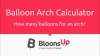Join BloonsUp News and get your FREE balloon arch tutorial, extra tips, discounts & more!

# Balloon Arch Calculator: How Many Balloons for an Arch or Garland?

The first thing you need to enter into your balloon arch calculator is the total length of the balloon arch you're planning. This determines the number of balloons you'll need for your arch.

How do you get the total length? Keep reading! Or skip down to get a copy of my free spreadsheet that does the calculating for you.

Btw, you can also use these instructions when you want to know how many balloons you'll need for a balloon garland.

If you make a purchase through a link on my site, I may receive a small commission at no extra cost to you. I only recommend products that I believe to have good value (affiliate disclosure).Do you find this balloon arch calculator helpful? Pin it for later or share with a friend!

To figure out the size of the balloon arch you want to build, measure the height and width of the area where it will be displayed.

Use the following calculations to work out the approximate total length of your arch line or frame.

Fig. 1
To make an arch that's wider than it is tall:
width + height = approximate total length.

Fig. 2
For an arch that has the same height and width:
(1.5 x height) + width = approximate total length.

Fig. 3
For an arch that's taller than it is wide:
(2 x height) + width = approximate total length.Use these diagrams to calculate the total length of your balloon arch, depending on its height and width.

Next, you want to calculate how many balloons you need. This depends on the type of balloon arch or garland you have in mind and the size of the balloons.

## Balloon Arch Calculator: Single Row Arch or Garland

For a 'String of Pearls' arch (i.e a floating arch with a single row of balloons) you'll need about 3 x 11" balloons per meter (this translates into 0.91 x 11" balloons per foot).

Example: Let's say your arch is 6m (20') wide and 6.7m (22') high. As your arch is taller than wide, formula 3 from above applies to calculate the arch length:

(2 x 6.7m) + 6.0m = 13.4m + 6.0m = 19.4 m

So your arch is roughly 20m long, which means you need 60 x 11" balloons (20m x 3 balloons per meter).

## How Many Balloons Do You Need for a Clustered Arch or Garland?

If the length of the balloon arch or garland and the diameter of balloons are in the same units (both in ft, in, m, cm, mm ) you can use this formula for an arch with clusters of 4 balloons:

Divide the diameter of the balloon into the length of the arch and multiple by 4.8 to get the number of balloons needed (thanks to Sean O'Kelly from balloondecor.com.au who shared this formula with our readers).

Length of arch (unit)
--------------------------------                        x     4.8 = number of balloons
Diameter of balloon (same unit)

Example:
You want to make an arch that is 6.1m (20') wide and 3.7m (12') high. You plan to use 11" balloons (28cm diameter). As your arch is wider than tall, formula 1 from above applies. We'll calculate everything in cm to stay with the same unit.

Arch length: 610cm + 370cm = 980cm

Number of balloons: 980cm / 28cm * 4.8 = 168 balloons

Number of 4 balloon clusters: 168 / 4 = 42

If you calculate everything in feet and inches, here's an easier way to estimate the number of balloons you need for a 4 balloon per cluster arch...

• 5 inch balloons = 12 per foot
• 9 inch balloons = 8 per foot
• 11 inch balloons = 6 per foot
• 14 inch balloons = 4 per foot
• 16 inch balloons = 4 per foot

Is your head spinning from all these numbers and formulas? Fear not. I've calculated the number of balloons for various arch or garland lengths for you.

## Balloon Arch / Garland ChartFind this chart helpful? Pin it for later or share with a friend.

Want to create beautiful balloon arches or garlands yourself, hassle-free and at an affordable cost? Check out my review of the five best DIY balloon arch kits.

## Free Balloon Arch Calculator For You

What if you could simply enter width and height of your arch, and the total arch lengths as well as number of balloons are "auto-magically" calculated for you?

That's exactly what my balloon arch calculator spreadsheet does. Watch the quick demo below and then get your free copy